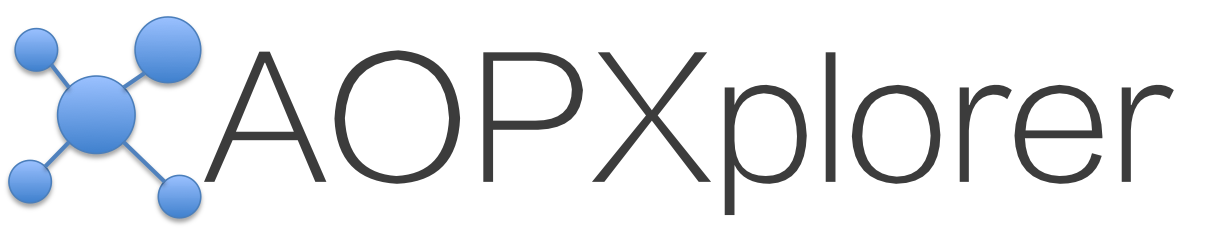Driving Biological Understanding of Data By Coupling Adverse Outcome Pathway Networks, R and Cytoscape

You've got AOPXplorer installed, let's run some examples.

### Example 1: Building an AOP Network (AOPN) for Steatosis

The AOPXplorer uses the AOP Ontology (AOPO) as its primary data source for AOPs. The AOPO is a community resource that is updated frequently to bring in the latest AOP knowledge from the community.

In this example, we will build an AOPN for Steatosis. There are several AOPs for steatosis in the AOPO. What we're going to do is bring all of these together into a single network. The good news is that the AOPO does most of the work for us -- it makes sure that the individual parts that are the same across the AOPs are brought together seamlessly. So, here goes:

First, we're going to load up the AOPXplorer library. Then we're going to use the get_aopn function to get only the "Steatosis" AOPN. Finally, we're going to print it out.

R Code:
library(aopxplorer)
x <- get_aopn("Steatosis")
plot(x)

### Example 2: Listing all of the adverse outcomes

The AOPO contains many different adverse outcomes (AOs). If you'd like to see the list of the AOs, you can easily query for them.

R Code:

### Example 3: Adding expression data to the networks

Let's say you have assay data that you want to overlay onto the network in Cytoscape. No problem. Just make sure you've got it as a list, and you're off to the races (Example 4 will cover a slightly more complicated situation to overlay data). For this example, you need to have data in your list for each node. When you run the V(x)\$name you'll get the listing of the node names in order. Make sure that your data list matches this order. So that your_data[] contains the data for PPARalpha. Keep in mind, if you had your data in a data.frame or something like that this would've been considerably easier. You could just slice and dice it, re-arrange, and put it into the proper order.

R Code:
x <- get_aopn("Steatosis")
V(x)\$name
your_data <- list()
your_data[] <- c(1,1,1,1)
your_data[] <- c(11, 12, 13, 14)
your_data[] <- c(15.4, 1.1, 17.2, 4.1)
your_data[] <- c(NA, NA, NA, NA)
your_data[] <- c(NA, NA, NA, NA)
your_data[] <- c(NA, NA, NA, NA)
your_data[] <- c(NA, NA, NA, NA)
your_data[] <- c(NA, NA, NA, NA)
your_data[] <- c(NA, NA, NA, NA)
your_data[] <- c(NA, NA, NA, NA)
your_data[] <- c(NA, NA, NA, NA)
data_steatosis_net <- associate_data_aopn(your_data, x)
cytoscape_aopn <- toCytoscape(data_steatosis_net)
send_aopn(cytoscape_aopn)

### Example 4: Adding expression data to the networks -- redux

Okay, so you've done Example 3 and you said, "well that's too much work!" Yeah, we agree. So let's try for something that's a little less work, a little more code-y, and hopefully not as manual. Let's imagine you have your data in a data.frame. Let's also imagine that you have data for more nodes than you have in the network. The first part of this example is going to build out the data so that we have something kinda realistic. The next part will do the fun part.

So let's first build the data (ordinarily, you'll already have this readily available to you) and we'll call it mat_dat. For these purposes, it's a matrix, but it could just as easily be a data.frame. All the steps after that are going to be things that you may need to do on a routine basis:

R Code:
mat_dat <- matrix(runif(60), nrow=15, ncol=4, byrow=TRUE, dimnames = list(c("PPARalpha", "HSD17B4", "blahx", "FXR", "steatosis", "blahy", "SREBP1", "Rheb1", "mTORC1", "IRS1", "Tsc1", "GSK3", "blahq", "blahs", "Akt2"), c("C1", "C2", "C3", "C4")))
x <- get_aopn("Steatosis")
V(x)\$name
mat_names_match <- rownames(mat_dat)[which(rownames(mat_dat) %in% V(x)\$name)]
mat_dat_pared <- mat_dat[mat_names_match, ]
mat_dat_pared_rearranged <- mat_dat_pared[match(V(x)\$name, mat_names_match),]
mat_dat_pared_list <- setNames(split(mat_dat_pared_rearranged, seq(nrow(mat_dat_pared_rearranged))), rownames(mat_dat_pared_rearranged))
data_steatosis_net <- associate_data_aopn(mat_dat_pared_list, x)
cytoscape_aopn <- toCytoscape(data_steatosis_net)
send_aopn(cytoscape_aopn)